### Score Statistics and Tests

To understand the general form of the score statistics, let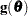be the vector of first partial derivatives of the log likelihood with respect to the parameter vector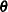, and let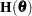be the matrix of second partial derivatives of the log likelihood with respect to. That is,is the gradient vector, andis the Hessian matrix. Let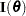be either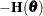or the expected value of. Consider a null hypothesis. Let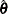be the MLE ofunder. The chi-square score statistic for testingis defined by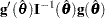It has an asymptotic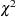distribution withdegrees of freedom under, whereis the number of restrictions imposed onby.